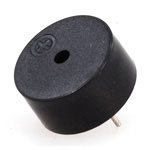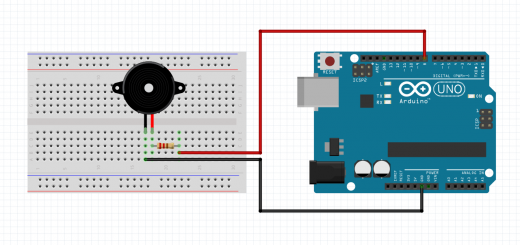Programming the AVR Timers¶

Read time: 25 minutes (6354 words)

Let’s explore some “C” code and see if we can create a simple signal that can drive a Piezo buzzer.

The Piezo Buzzer¶

• Here is the buzzer we will use• a crystal inside of this flexes when a voltage is applied across the leads
• when it flexes, it pushes air out of the way.
• Flex it fast enough and those air pulses hit your ear making noise
• You control the tone by the pulse rate

Buzzer Code¶

• Here is the start of our code
// Example Arduino code to sound a Piezo buzzer

#include <avr/io.h>
#include <util/delay.h>

#define CLOCK_PRESCALE(n)   (CLKPR = 0x80, CLKPR = (n))
#define BUZZER_CONFIG       (DDRB |= (1<<4))
#define BUZZ_ON             (PORTB |= (1<<4))
#define BUZZ_OFF            (PORTB &= ~(1<<4))

This code sets up the chip clock so it runs at a slower than full speed. There are defines that set up the output pin and toggle that pin on and off. We will use these macros in the main code.

Chip setup¶

Next we configure the chip

int main(void){
CLOCK_PRESCALE(0);
BUZZER_CONFIG;

We are going to connect the buzzer between Arduino pin 12 (PORTB bit 4) and the one of the ground lines on the opposite side of the board.

The buzzer loop¶

Finally, we enter the main loop

while(1) {
BUZZ_ON;
_delay_us(200);
BUZZ_OFF;
_delay_us(200);
}

Connecting the buzzer¶

Here is how to hook up a simple Piezo buzzer to output pin 12 on the Arduino board (PB4 on the chip itself).Note

This image shows the buzzer connected to another pin on the Arduino. Any output pin will do, you just need to modify the configuration definitions to make it work.

Here is a picture of the chip, with all of the pins identified. This will help in hooking up other stuff to the board:What is wrong with this?¶

Running this code makes noise (it did on my system, but not very loud!)

The program works, but it consumes all the processor’s attention That wastes power we could use for other purposes. One way to solve this is to us an internal Timer.

Warning

There is one more problem with this code: IT WILL NOT SHUT UP! The code runs forever, generating an annoying tone. At least we know it works!

A Timer is just a simple counter that cab be set up and let run. The timer counts using the system clock. Once the timer reaches a max value, it rolls over and we can detect that!

AVR Timers¶

The chip on the Arduino has four internal timers that go by these names:

• Timer0 - 8 bit counter
• Timer1 - 16 bit counter
• Timer3 - 16 bit counter
• Timer4 - 10 bit counter

Hmmm, wonder what happened to Timer2?

Clock source¶

We mentioned that the timer counts using the system clock. To give more control of the timing we can divide that system clock using something called a prescaler to extend the time it takes reach the max count value.

The possible prescaler settings available on these timers are:

• Clock / 8
• Clock / 64
• Clock / 256
• Clock / 1024

Timer counting rates¶

On the Arduino, we can configure timers to increment the counter every:

• 8/16000000 = 0.5us
• 64/16000000 = 4us
• 256/16000000 = 16us
• 1024/16000000 = 64us

Setting the timer count¶

Each timer has a fixed number of bits available for its counting register. That means they can count up to:

• 255 for an 8-bit timer
• 1024 for a 10-bit timer
• 65536 for a 16-bit timer

We can detect when the timer “rolls over” from the max value it can hold to zero. That event can signal an interrupt, or simply set a flag we can check in our code. That is called “polling”.

The timers begin operation when we load a count into their counting register. If we start the timer with zero, it will rake time to reach the maximum count. If we choose to load some value other than zero in the counter, the time it takes to “roll over” will be shorter.

Maximum timer times¶

We will be using the 8-bit Timer0 timer for this experiment. With the available prescallar values, we cna get these times out of the timer:

• 256 * 0.5us = 128us = 7812Hz
• 256 * 4us = 1.024ms = 976Hz
• 256 * 16us = 4.096ms = 244Hz
• 256 * 64 = 16.384ms = 61Hz

Of course, we can adjust these numbers. We also have control over how fast the main clock ticks, usina another scaling register that divides down the main system oscillator.

Detecting the roll over¶

We can deal with the signal one of two ways:

• write code that checks for roll over every so often (polling)
• configure the chip to generate an interrupt when it happens

For this experiment, we will use “polling”.

Configuring the Timer¶

In order to use a timer, we must set it up and start it running. There are two tasks we need to do:

• set the prescaler value
• preload the counter

For Timer0, the control registers we use to control the count rate is:

• Timer/Counter Control Register B - TCCR0B

• Bit 0 = CS0
• Bit 1 = CS1
• BIT 2 = CS2

Setting the prescaler¶

 CS2 CS1 CS0 Function 0 0 0 Timer stopped 0 0 1 clock/1 0 1 0 clock/8 0 1 1 clock/64 1 0 0 clock/256 1 0 1 clock/1024

Setting the preload count¶

THe register Timer0 uses for counting is:

• Timer/Counter0 count register - TCNT0

• This register is set with an initial counter value.
• Setting the count starts the timer (if enabled)

Detecting the roll over¶

Each timer has a bit that is set whenever the timer overflows. For Timer0 thise bit we will use is this:

• Timer Interrupt Flag Register - TIFR0
• The bit we will examine is TOV0 (overflow).

Polling code¶

We will write the polling code in assembly language. THis file looks like others we have set up, except that we need to make the single function in this file global`. THe “C” code will reference this function to do the delay. In this example, we are not passing any data between “C” and assembly language code. Doing that is a topic for later.

Here is the timer code. This function configures the timer, starts it up for the delay time, then shuts it down when done.

timer.S
 1 2 3 4 5 6 7 8 9 10 11 12 13 14 15 16 17 18 19 #include "config.h" .section .text .global timer_delay timer_delay: out _(TCNT0), r1 ; zero counter ldi r24, 0x3c ; set compare value out _(OCR0A), r24 ldi r24, (1 << WGM01) ; CTC max trigger out _(TCCR0A), r24 ldi r24, (1 << CS02) | (1 << CS00) ; clk/1024 out _(TCCR0B), r24 1: sbis _(TIFR0), 1 ; (1 << TOV0) ; check interrupt flag rjmp 1b out _(TCCR0B), r1 ldi r24, (1 << OCF0A) out _(TIFR0), r24 ret

Skipping instructions¶

Some AVR instructions are a bit funny. In this code you will see the sbis instruction:

• Test some condition (bit in register is set)
• If the condition is met, skip the next instruction
• Otherwise, they execute the next instruction
• Usually this is a jump of some kind

The result is like our conditional branch, but it takes two instructions

Is this better?¶

With this code, the processor is not really stuck in a loop until the overflow bit gets set, even though that is how we set this delay function to work. We could be off doing other things until that flag bit gets set. This is sure easier to control that that ugly nested counting loop we used earlier!

Rewriting the Code¶

We will set up this project in a new folder with a single Makefile. The Makefile shown here can build any project involving C or AVR Assembly code:

Makefile
 1 2 3 4 5 6 7 8 9 10 11 12 13 14 15 16 17 18 19 20 21 22 23 24 25 26 27 28 29 30 31 32 33 34 35 36 37 38 39 40 41 42 43 44 45 46 47 48 49 50 51 52 53 54 55 56 57 58 59 60 61 # source files TARGET := $(shell basename$(PWD)) CSRCS := $(wildcard *.c) COBJS :=$(CSRCS:.c=.o) SSRCS := $(wildcard *.S) SOBJS :=$(SSRCS:.S=.o) OBJS := $(COBJS)$(SOBJS) LST := $(TARGET).lst # define the processor here MCU := atmega328p FREQ := 16000000L # define the USB port on your system #PORT := /dev/ttyACM0 PORT := /dev/tty.usbmodem14101 PGMR := arduino # tools GCC := avr-gcc OBJDUMP := avr-objdump OBJCOPY := avr-objcopy DUDE := avrdude UFLAGS := -v -D -p$(MCU) -c$(PGMR) UFLAGS += -P$(PORT) UFLAGS += -b115200 CFLAGS := -c -Os -mmcu=$(MCU) CFLAGS += -DF_CPU=$(FREQ) LFLAGS += -mmcu=$(MCU) .PHONY all: all:$(TARGET).hex $(LST) # implicit build rules %.hex: %.elf$(OBJCOPY) -O ihex -R .eeprom $<$@ %.elf: $(OBJS)$(GCC) $(LFLAGS) -o$@ $^ %.o: %.c$(GCC) -c $(CFLAGS) -o$@ $^ %.o: %.S$(GCC) -c $(CFLAGS) -o$@ $< %.lst: %.elf$(OBJDUMP) -C -d $< >$@ # utility targets .PHONY: load load: $(DUDE)$(DUDECONF) $(UFLAGS) -Uflash:w:$(TARGET).hex:i .PHONY: clean clean: \$(RM) *.hex *.lst *.o *.elf

main.c: Setting up¶

We start off by writing the configuration file we will use for this project:

config.h
 1 2 3 4 5 6 7 8 9 #include #define BUZZ_PIN 4 #define BUZZ_DIR DDRB #define BUZZ_PORT PORTB #define _(s) _SFR_IO_ADDR(s)

We will set this project up a bit differently. This one will use a standard “C” main function that handles the basic buzz logic. We will use Timer0 in compare match mode to do the delay logic. That routine will be written in assembly language.

main.c
 1 2 3 4 5 6 7 8 9 10 11 12 13 14 15 16 17 18 19 20 21 22 23 24 25 26 #include "config.h" extern void timer_delay(); #define BUZZ_ON (BUZZ_PORT |= (1<

How it works¶

• Main sets up the chip and timer system

• It then loops toggling buzzer pins and calling the timer delay routine
• timer.S configures the timer timer
• It uses polling in the delay loop to wait for the timer overflow
• Overall logic is identical to the “C” example

Examing the Code¶

The Makefile used in this project creates a listing file (with en extension of .lst). This file is a “disassembly” of the final binary code sent to the processor. Eamining this file is how I discovered the problems with the current linker. I am still working on figuring out how to control the link step so I can generate pure assembly language files properly!